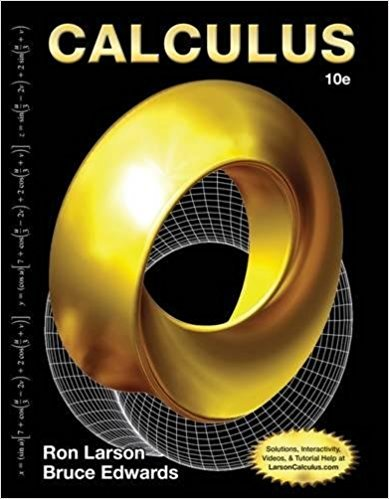×
×

# Solutions for Chapter 4: Calculus 10th Edition## Full solutions for Calculus | 10th Edition

ISBN: 9781285057095Solutions for Chapter 4

Solutions for Chapter 4
4 5 0 234 Reviews
18
2
##### ISBN: 9781285057095

Since 20 problems in chapter 4 have been answered, more than 2705 students have viewed full step-by-step solutions from this chapter. This expansive textbook survival guide covers the following chapters and their solutions. Chapter 4 includes 20 full step-by-step solutions. This textbook survival guide was created for the textbook: Calculus, edition: 10. Calculus was written by and is associated to the ISBN: 9781285057095.

Key Calculus Terms and definitions covered in this textbook
• Additive inverse of a real number

The opposite of b , or -b

• Arcsecant function

See Inverse secant function.

• Central angle

An angle whose vertex is the center of a circle

• Elementary row operations

The following three row operations: Multiply all elements of a row by a nonzero constant; interchange two rows; and add a multiple of one row to another row

• Focus, foci

See Ellipse, Hyperbola, Parabola.

• Heron’s formula

The area of ¢ABC with semiperimeter s is given by 2s1s - a21s - b21s - c2.

• Law of cosines

a2 = b2 + c2 - 2bc cos A, b2 = a2 + c2 - 2ac cos B, c2 = a2 + b2 - 2ab cos C

• Linear equation in x

An equation that can be written in the form ax + b = 0, where a and b are real numbers and a Z 0

• Probability function

A function P that assigns a real number to each outcome O in a sample space satisfying: 0 … P1O2 … 1, P12 = 0, and the sum of the probabilities of all outcomes is 1.

• Projection of u onto v

The vector projv u = au # vƒvƒb2v

A function that can be written in the form ƒ(x) = ax 2 + bx + c, where a, b, and c are real numbers, and a ? 0.

• Quartile

The first quartile is the median of the lower half of a set of data, the second quartile is the median, and the third quartile is the median of the upper half of the data.

• Real number line

A horizontal line that represents the set of real numbers.

• Reciprocal of a real number

See Multiplicative inverse of a real number.

• Resistant measure

A statistical measure that does not change much in response to outliers.

• Spiral of Archimedes

The graph of the polar curve.

• Square matrix

A matrix whose number of rows equals the number of columns.

• Symmetric difference quotient of ƒ at a

ƒ(x + h) - ƒ(x - h) 2h

• y-intercept

A point that lies on both the graph and the y-axis.

• Zero factor property

If ab = 0 , then either a = 0 or b = 0.

×